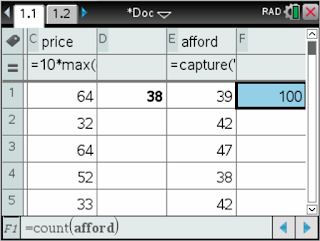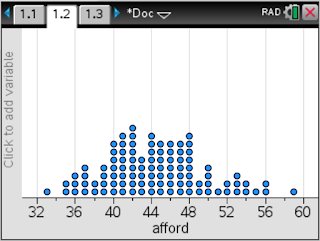## Saturday, June 19, 2021

### My Google Data Analytics Capstone Project

What do you hope to take away from this capstone project?
I am hoping to show that I can do it, and maybe demonstrate a practical understanding of data dashboards so I can better serve the schools I am working with.

What is one important skill you think you’ll learn?
I think just brining it all together...R, Kaggle, Tableau...

Which skills do you most look forward to demonstrating?
Google Data Studio perhaps? But I have learned a few new google sheets tricks

What are some issues you might encounter?
Having my OCD kick in.

## Sunday, October 4, 2020

Over the course of 100 or 1,000 or 10,000 flips, will there be more heads or more tails? And once one side of the coin takes the lead, do they relinquish it at some point?

I explored this with a simulation using Texas Instruments' Nspire CX CAS with Python.

How many coin flips does it take to get N heads in a row? Here is a simulation in Texas Instruments' Nspire CX CAS with Python

### Messing with Python on the TI Nspire CX CAS

A reverse raffle is where you purchase a raffle ticket, and all the tickets are placed in a large bucket, and the last ticket pulled out is the winner.

I used to do a variation of a reverse raffle for grading purposes. I would number my groups, say, 1 through 8, and then randomly choose numbers on the Nspire until only one number was left. My twist was that if a number was chosen, if it is still in play, you remove it. If the number has been removed, put the number back in play.

I wrote a nice python script to do this in Trinket.io

Now that @TICalculators has introduced a sweet Python implementation on the Nspire, I created the script there:

## Saturday, May 25, 2019

### Teaching factoring without teaching factoring

I haven't taught Algebra 1 in a few years. But I was motivated to write something down in response to this thread, and in particular, Benjamin Dickman's tweet:

In particular, this.
"but I'm confident there's a way to broach factoring trinomials consonant with [e.g. youcube's description of] number sense."
I think I had a way to broach factoring trinomials that promotes number sense.

Consider this in the context of teaching kids how to multiply binomials and trinomials and such. I would emphasize the distributive property in conjunction with the area model for students who needed a bit of structure or organization. I also made a conscious effort to make connections to multiplying integers.

I also would introduce students to dividing a trinomial by a binomial. This was a natural extension to what we were doing, and the question "Can we only multiply, or can we divide, too?" seemed to always come up.

This probably took the better part of three to four weeks (so about 15 instruction days.)

Every day, in anticipation of introducing the idea of factoring after working on the idea of multiplying and dividing polynomials, I would provide my students with a short number puzzle or two as a starter problem or bell ringer or whatever you would call it. The puzzles would be along the lines of something like this:
Find two numbers whose product is 18 and whose sum is 11.
This is the same question students ask themselves when they are asked to factor $x^2+11x+18$.

I would often provide two puzzles that would complement each other. Possible second puzzles to accompany the one one above might be:
Find two numbers whose product is 18 and whose sum is -9.
or

Find two numbers whose product is -18 and whose sum is 7.
Again, these are the questions kids would ask themselves when factoring $x^2-9x+18$ and $x^2+7x-18$, respectively.

When I would introduce students to the patterns arising from multiplying squaring binomials my number puzzle would be:,
Find two numbers whose product is 25 and whose sum is 10.
When I would introduce students to the patterns arising from multiplying conjugate binomials such as $x^2-5$ and $x^2+5$, my number puzzle would be:
Find two numbers whose product is -25 and whose sum is 0.

Needless to say, the kids became incredibly proficient in solving these number puzzles and creating their own for others to try. Now is the time to throw them a challenge:
Find four numbers $a, b, c,$ and $d$ where $ac=3$ and $bd=5$ and $ad+bc=16$.
This might be the question kids would ask themselves when factoring $3x^2+16x+5=(3x+1)(x+5)$

One day, the kids would come in, and their starter puzzle would be:
Two binomials were multiplied and the resulting product was $x^2+8x+12$. What are the two binomials that were multiplied together?
So after a month of number puzzles each day to start class, factoring became just another number puzzle. As the solution to the puzzle would percolate through the room, the realization was that this is just another form of the number puzzles we had been doing.  I would spend less than two weeks on factoring, because the kids had been factoring through number puzzles for a month by then.

## The problem.

This problem was introduced to me by Allan Rossman at the Simulating at Serenbe workshop last summer. The problem goes like this...

Suppose that you have only 50 cents in your pocket and you want to buy an ice cream cone.  The owner of the ice cream shop offers a random price determined as follows: You roll a pair of fair, six-sided dice, and the price is the larger number followed by the smaller number (in cents).  We will approximate, and then determine, the probability that you’ll be able to afford the ice cream cone.

Allan used a three-step approach to these problems that I would like to incorporate into as much as I can in stats. His approach to these problems involved intuition, simulation, and mathematics. Begin by getting a feel for the problem and the probability via your intuition (which is often wrong), then set out to conduct some sort of a simulation to get a better feel for the probability (Allan's choice was R, but I will share a GeoGebra simulation and an Nspire simulation and program), then tackle the mathematics of computing the probability (which in this problem, is more of listing all the outcomes in the sample space).

### Intuition

Before you jump into the problem, think about some specific prices that you could possibly pay for a cone. You could pay 43 cents when the owner rolls a 4 and a 3. How likely is this? What about paying 21 cents? How likely would it be to pay 23 cents (trick question)? What's the largest amount you would be asked to pay (regardless if you can afford it)? What's the smallest amount?

### Simulation

#### TI NspireCAS Simulation

I would want my students to actually roll some dice to begin with as a transition from intuition to simulation. Roll some dice, create some dot plots by hand as a class. I would then help them set up a spreadsheet on their Nspires. Since every AP Stats student will be loaned a TI NspireCAS this year (quite excited), most of the stat stuff you will see will involve the Nspire. If my school buys it, I am going to use it.

Here is a spreadsheet simulating 100 rolls of the dice, computing the price, and counting the number of prices less than 50 cents.Randint(1,6,100) to generate 100 random rolls of an imaginary die.10*max(die1,die2) + min(die1,die2) computes the price for the two rolls

Here is a distribution of 100 simulated prices.100 prices by 100 simulated rolls of the dice.

I am interested in how many prices are less than 50 cents, so I will keep track of this in another cell in the spreadsheet.countif(price,?<50) counts the number of values in the price column that are less than 50.

By storing this number, I can use automatic data capture to insert these values into another list.capture(aff,1) allows me to automatically capture the number of prices less than 50 in a list when I press ctrl+r on the handheld

I can then generate a list of the number of prices out of each 100 simulations that are less than 50 cents.count(afford) allows me to keep track of how many trials I have run. There are 100 data values in the afford list.

A dot plot of these numbers somewhere between 40 and 48 times out of 100 I will be able to afford an ice cream cone.Here are the number of prices that I can afford out of 100 simulations of 100 simulated rolls of the dice.

As soon as the students ask, "Is there a way to just press ctrl+r and have it do all this at one time?" they are ready to be introduced to writing a simple TI-Basic program that runs this simulation.

#### GeoGebra Simulation

Here is a GeoGebra simulation of rolls of the dice. This is pretty straight forward.

#### Desmos Activity Builder

Last week I was invited to attend the Desmos Fellows Weekend. Spending the weekend out there really got me going with the Desmos Activity Builder and the Computation Layer. Here is a Desmos Activity based upon this problem:  https://student.desmos.com?prepopulateCode=tjbeah

One of my goals for this year (besides trying to blog again) is to create Desmos Activities that accompany the Advanced Quantitative Reasoning text I use with some of my Seniors who are not in AP Stats.

### My Google Data Analytics Capstone Project

What do you hope to take away from this capstone project? I am hoping to show that I can do it, and maybe demonstrate a practical understand...